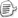# 一、PTA实验作业（5分）

## 题目1：6-8 使用函数实现字符串部分复制

### 1. 本题PTA提交列表（要提交列表，不是结果）### 2. 设计思路（伪代码或流程图）

• 伪代码：
  函数定义void strmcpy( char *t, int m, char *s )
定义i，n两个变量
for i=0  to  *(t+i)!='\0'  i++
end
n=i  //用循环记录字符的长度
判断输入的m是否小于长度n，若是进入循环｛｝
for i=0  to  *(t+m+i-1)!='\0'  i++  ｛
*(s+i)=*(t+m+i-1);
end ｝
*(s+i)='\0';      ｝
若m大于n则
*s='\0';

• 流程图：### 3.代码截图（注意，截图，截图，截图。不要粘贴博客上。不用用···语法去渲染）### 4.本题调试过程碰到问题及PTA提交列表情况说明。

• 首先我遇到的情况是m=n时我没有将它考虑进去，我直接判断了m<n的情况，如上图所示。
• 还有就是我在判断从第m为开始输出时我是直接p+m+i，其实是要p+m+i-1，因为数组是从0开始的。

## 题目2：6-9 求子串在母串中最后一次出现的地址

### 1. 本题PTA提交列表（要提交列表，不是结果）### 2. 设计思路（伪代码或流程图）

   定义函数char *fun (char *s, char *t ){
定义n,i,j,flag=0,l 5个变量
for  i=0  to  *(s+l)!='\0' {
for(i=l,j=0;*(s+i)==*(t+j);i++,j++) {
如果子串到达结束符位置即*(t+j+1)=='\0 '   {
flag=1; n=l;  } //flag 做为出现子串的标志
end}
end }
如果没有子串即flag==0
返回   NULL;
否则
返回  s+n;
}



### 3.代码截图（注意，截图，截图，截图。不要粘贴博客上。不用用···语法去渲染）v

### 4.本题调试过程碰到问题及PTA提交列表情况说明

• 这道题刚开始我竟然忘记写第一个循环了，导致一直错误，因为在课上老师讲过这道题所以
我就翻看了以前的题目改了过来，还有编译错误的原因是我在写*t+j+1时忘记加括号了这是
一个失误点。

## 题目3：6-10 字符串串动变化

### 1. 本题PTA提交列表（要提交列表，不是结果）### 2. 设计思路（伪代码或流程图）

 定义函数void * fun(char *p){
定义变量 int n,i
max=*p; //存储第一个字符
for i=1 to  *(p+i)!='\0'{
比较每一个字符找出其中最大值放入max中
end}
x=*(p+n);//先储存第n个数防止移位后改变
for(i=n;i>0;i--){
*(p+i)=*(p+i-1);//将字符移到后一位
end}
*p=x //再将原先储存的内容赋值于第一位


### 3.代码截图（注意，截图，截图，截图。不要粘贴博客上。不用用···语法去渲染）### 4.本题调试过程碰到问题及PTA提交列表情况说明。

• 这道题主要错在我移位之后导致数组第n个字符已经改变，而我没有给他先保存下来
导致赋给数组第一个时是改变后的值，导致错误。

# 二、截图本周题目集的PTA最后排名。（2分）# 三、阅读代码（2分）

## 题目1：## 优秀解答：

#include <stdio.h>

void main(){
long int digit;		//存放结果的数组,0号元素放整数部分,其余放小数部分
long int remainder;   //存放余数
int state=0;	//是否是循环小数,默认不是
long int repetendstart=0;	//循环节的开始位置和结束位置
long int n,d;	//输入的分子和分母
int i;
printf("input N/D:");
scanf("%d/%d",&n,&d);	//输入分子和分母

digit=n/d;
remainder=n%d;	//求出第一个余数
i=0;
while(remainder[i] && !state && i<100){ //求小数部分
i++;        //i记录了求了多少位小数
digit[i]=remainder[i-1]*10/d;	//求出一位小数
remainder[i]=remainder[i-1]*10%d;	//求余数即下一次的被除数
for(int j=0;j<i;j++){	//判断是否出现循环节
if(remainder[j]==remainder[i]){//如果出现循环节则记下节开始的位置
repetendstart=j+1;
state=1;	//置是循环小数状态
break;
}
}
}

//以下是打印部分
printf("%d",digit);	    //打印整数部分
if(remainder!=0) printf(".");    //如有小数则打印小数点
for(int j=1;j<=i;j++){      //打印小数部分
if(j==repetendstart) printf("(");
printf("%d",digit[j]);
}
if(state) printf(")");
printf("\n");
}

• 这题难点在于如何求出循环的小数部分，如何在输出时用括号括起来。
• 我找到的方法是用两个数组，一个储存整数部分，一个储存小数部分，因为只有分开去才可以判断出循环的部分，
在求小数的部分时加入内循环判断是否出现循环的小数，就是加入一个循环，而难点也在这里，我看了好久，主要原
理是在内循环中找到一个位置使其与现在的被除数相同，然后在加上1就是循环小数开始的位置，记录下这个位置，
直接退出循环，不仅求出了循环体小数的位置，且可以直接退出，执行输出。

## 题目2：## 优秀解答：

void CountOff( int n, int m, int out[] ){
int i=0,j=0,k=0,cnt=0,a[MAXN];

for(i=0;i<n;i++)  //先对应的人的报数赋值
a[i] = i+1;

i=0;
while(cnt < n){
if(a[i]!=0)
k++;  //记录报数的位置
if(k==m){
j++;
out[i]=j;  //第几次退出
k=0;  //重新开始
cnt++;  //剩余的人
a[i]=0;  //使其为0跳过
}

i++;
if(i==n)//遇到最后一位从0开始
i=0;
}  }

• 这道题刚开始连题目都看不懂，我以为是输出退出的第几个人，但是题目却是4 10 1 7 5 2 11 9 3 6 8，我还以为是
题目错了，后来才知道是输出退出的是第几次。
• 首先，是先把报数的人赋值为1~n，k记录报数的数值，直到报到m，然后out[i]=j，j是指第几次输出，在重新赋值，
知道最后一个数字都退出。这里用2个数组直接就作出了，一个用来判断报数的人，一个来记录退出的是第几次。

# 四、本周学习总结（1分）

## 1.自己总结本周学习内容。

• 学会了指针在函数中的传参，同时也学会了用指针来指向数组，如*（a+i）
• 同时也知道*a++是错的*a+i必需加上括号。
• 还有就是用二维数组来存储多个字符，如输入多个字符串可以定义char a[][],行输入第几个数组，列输入字符串。
• 另外指针的赋初值，如果忘记给指针赋值的话就会导致程序崩溃。
• 学会了string的库函数的用法，如字符的连接strcat，字符串的复制strcpy等等

## 2.罗列本周一些错题。• 这题就是我用*n++导致错误。• 这题要求输出的是整数的值，答案应该是sum=sum+*s-'0'而我却忘记减0了。posted on 2017-12-16 18:33  陈张鑫  阅读(237)  评论(7编辑  收藏  举报